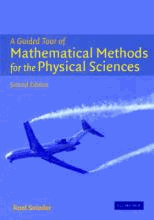# A Guided Tour of Mathematical Methods : For the Physical Sciences by Snieder, Roel

• R 400.00
Unit price per
• Save R 891.58
Tax included.

ISBN: 9780521834926

CAMBRIDGE UNIVERSITY PRESS | 15 October 2004

Hardback | 522 pages

Mathematical methods are essential tools for all physical scientists. This second edition provides a comprehensive tour of the mathematical knowledge and techniques that are needed by students in this area. In contrast to more traditional textbooks, all the material is presented in the form of problems. Within these problems the basic mathematical theory and its physical applications are well integrated. The mathematical insights that the student acquires are therefore driven by their physical insight. Topics that are covered include vector calculus, linear algebra, Fourier analysis, scale analysis, complex integration, Green's functions, normal modes, tensor calculus and perturbation theory. The second edition contains new chapters on dimensional analysis, variational calculus, and the asymptotic evaluation of integrals. This book can be used by undergraduates and lower-level graduate students in the physical sciences. It can serve as a stand-alone text, or as a source of problems and examples to complement other textbooks.